# IBPS Clerk Mains Quantitative Aptitude Questions 2019 (Day-21) High Level New Pattern

Dear Aspirants, Quantitative Aptitude plays a crucial role in Banking and all other competitive exams. To enrich your preparation, here we have provided New Pattern Aptitude Questions for IBPS Clerk Mains. Candidates those who are going to appear in IBPS Clerk Mains can practice these questions daily and make your preparation effective.

[WpProQuiz 4940]

Direction (1 – 5): Study the following information carefully and answer the given questions:

The table shows percentage of work done by each persons and time taken (Days) by each person to do that work.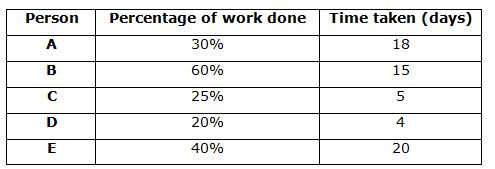1) A and B working alternatively starting with A and work for 28 days after that both are left and then the remaining work completed by C and E. If C and E work alternatively starting with C then how many days remaining work will be completed?

a) 17(1/2) days

b) 18(1/2) days

c) 20(1/2) days

d) 13(1/2) days

e) None of these

2) If D work with 25 % more efficiency for 4 days after that D left and then for 12 days B work with 40 % less efficiency and A work with 50% more efficiency together. Then the remaining work completed by another person F in 15 days. Find in how many days F can complete the whole work alone?

a) 120 days

b) 180 days

c) 140 days

d) 160 days

e) None of these

3) If E work with 25% more efficiency to finish the work and F takes 75% of the days which E takes to finish 125% of work. Efficiency of G is twice of E, in how many days will the work be completed if E is worked with F and G on alternative days starting with F?

a) 16(1/8) days

b) 14(1/8) days

c) 7(1/8) days

d) 9(1/8) days

e) None of these

4) Five persons A, B, C, D and E work alternatively in such a way that, on first day, A and B work together and on second day C, D and E work together, find in how many days whole work will be completed?

a) 11(1/2) days

b) 10(1/2) days

c) 12(1/2) days

d) 9(1/2) days

e) None of these

5) A, B, C and E completed a piece work costing Rs. 40600. If their daily wages are in the ratio of 5 : 7 : 12 : 6, then what amount will be received by A and E together?

a) Rs. 24000

b) Rs. 30000

c) Rs. 25000

d) Rs. 28000

e) None of these

Directions (6 – 10): The questions below are based on the given Series-I. The series-I satisfy a certain pattern, follow the same pattern in Series-II and answer the questions given below.

6)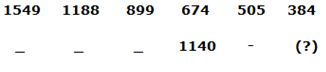a) 850

b) 900

c) 920

d) 825

e) 720

7)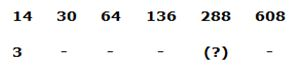a) 202

b) 196

c) 144

d) 112

e) 214

8)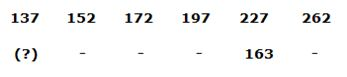a) 65

b) 73

c) 87

d) 53

e) 51

9)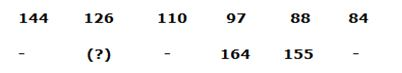a) 210

b) 187

c) 193

d) 171

e) 157

10)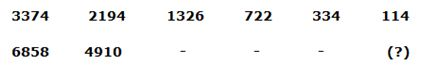a) 524

b) 612

c) 670

d) 718

e) 656

Directions (1-5):

Number of days A to complete the whole work alone=18*100/30=60 days

Number of days B to complete the whole work alone=15*100/60=25 days

Number of days C to complete the whole work alone=5*100/25=20 days

Number of days E to complete the whole work alone=20*100/40=50 days

A and B total unit of work = 300 unit

A complete the work in first day = 5 units

B complete the work in second day = 12 units

Total work completed after two days = 5 + 12 = 17 units

Both are working 14 and 14 days respectively

Work completed after 28 days = (5*14) + (12*14) = 238

Remaining work = 300 – 238 = 62

C and E total units = 100

First day complete C = 5 units

Second day complete E = 2 units

16 days = 5*8 + 2*8 = 56 unit complete after 16 days

Remaining unit = 6

17th day C complete 5 units of work

18th day E complete = 1/2 days

Total time = 16+1+1/2 = 17(1/2) days

Number of days D to complete the whole work alone = 4*100/20 = 20 days

D 25% more efficiency = 20*100/125 = 16 days

D Work for only 4 days = 4/16 = 1/4

Remaining work = 3/4

Number of days A to complete the whole work alone =18*100/30 = 60 days

A 50% more efficiency = 60*100/150 = 40 days

Number of days B to complete the whole work alone = 15*100/60 = 25 days

B 40% less efficiency = 25*140/100 = 35 days

A+B work for 12 days complete the work = 12*(1/40 + 1/35)

= 12* [(7+8)/280]

= 3 * [15/70]

= 9/14

Remaining work = ¾ – 9/14 = 3/28

F completed 3/28 work in 15 days.

Whole work completed by F = (28/3)*15 = 140 days

Number of days E to complete the whole work alone=20*100/40=50 days

E takes 25% more efficiency=50*(100/125) = 40days

F complete the whole work=40*(75/100)*(100/125) = 24 days

As efficiency of G is twice that of E so time taken by G = 20 days

LCM of (40, 24, 20) = 120

E complete = 3 units per day

F = 5 units

G = 6 units

First day work E and F together = 3 + 5 = 8 units

Second day E and G = 3 + 6 = 9 units

Two days work = 8+9 = 17 units

After 14 days work = 17*7 = 119 units

Remaining work = 1 unit, which is to be completed by E and F in 1/8 days

Total time = 14 + 1/8 = 14(1/8) days

Number of days A to complete the whole work alone=18*100/30=60days

Number of days B to complete the whole work alone=15*100/60=25 days

Number of days C to complete the whole work alone=5*100/25=20 days

Number of days D to complete the whole work alone=4*100/20=20 days

Number of days E to complete the whole work alone=20*100/40=50 days

LCM of (60, 25, 20, 20 and 50) = 300

A complete =5 units

B = 12 units

C = 15 units

D = 15 units

E = 6 units

First day work of A and B = 5 + 12 = 17 units

Second day work C, D and E = 15 + 15 + 6 = 36 units

First two days work = 17 + 36 = 53 units

First 10 days work = 53*5 = 265 units

Remaining =300 – 265 = 35

11th day = 17 units

Remaining work = 35 – 17 = 18 units

12th day = 18/36 = 1/2

Total work = 11(1/2) days

Number of days A to complete the whole work alone=18*100/30=60days

Number of days B to complete the whole work alone=15*100/60=25 days

Number of days C to complete the whole work alone=5*100/25=20 days

Number of days E to complete the whole work alone=20*100/40=50 days

Ratio of wages = 5*60 : 7*25 : 12*20 : 6*50

= 300 : 175 : 240 : 300 = 60 : 35 : 48 : 60

(A + E)’s wages = 40600*(120/203) = Rs. 24000

Directions (6-10):

Series I pattern:

1549 – 192 (361) = 1188

1188 – 172 (289) = 899

899 – 152 (225) = 674

674 – 132 (169) = 505

505 – 112 (121) = 384

Series II pattern:

2015 – 192 (361) = 1654

1654 – 172 (289) = 1365

1365 – 152 (225) = 1140

1140 – 132 (169) = 971

971 – 112 (121) = 850

Series I pattern:

14 * 2 + 2 = 28+2 = 30

30 * 2 + 4 = 60 + 4 = 64

64 * 2 + 8 = 128 + 8 = 136

136 * 2 + 16 = 272 + 16 = 288

288 * 2 + 32 = 576 + 32 = 608

Series II pattern:

3 * 2 + 2 = 6 + 2 = 8

8 * 2 + 4 = 16 + 4 = 20

20 * 2 + 8 = 40 + 8 = 48

48 * 2 + 16 = 96 + 16 = 112

112 * 2 + 32 = 224 + 32 = 256

Series I pattern:

137 + 15 = 152

152 + 20 = 172

172 + 25 = 197

197 + 30 = 227

227 + 35 = 262

Series II pattern:

73 + 15 = 88

88 + 20 = 108

108 + 25 = 133

133 + 30 = 163

163 + 35 = 198

Series I pattern: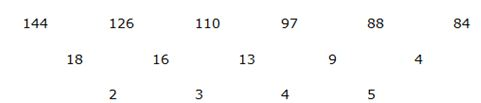Series II pattern: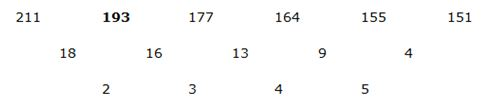Series I pattern:

153 – 1 = 3374

133 – 3 = 2194

113 – 5 = 1326

93 – 7 = 722

73 – 9 = 334

53 – 11 = 114

Series II pattern:

193 – 1 = 6858

173 – 3 = 4910

153 – 5 = 3370

133 – 7 = 2190

113 – 9 = 1322

93 – 11 = 718